Courses

# Types of Probability JEE Notes | EduRev

## JEE : Types of Probability JEE Notes | EduRev

The document Types of Probability JEE Notes | EduRev is a part of the JEE Course Mathematics (Maths) Class 11.
All you need of JEE at this link: JEE

G. CONDITIONAL PROBABILITY AND MULTIPLICATION THEOREM

Let A and B be two events such that P(A) > 0. Then P(B|A) denote the conditional probability of B given that A has occurred. Since A is known to have occurred, it becomes the new sample space replacing the original S. From this we led to the definition.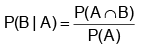which is called conditional probability of B given A. ⇒  P (A ∩ B) = P(A)   P(B | A) which is called compound probability or multiplication theorem. It says the probability that both A and B occur is equal to the probability that A occur times the probability that B occurs given that A has occurred.

Note : For any three events  A1, A2, A3 we have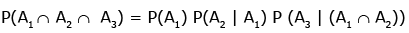Ex.13 Two dice are thrown. Find the probability that the numbers appeared has a sum of 8 if it is known that the second dice always exhibits 4.
Sol.
Let A be the event of occurrence of 4 always on the second die = {(1,4), (2, 4), (3, 4) (4,4), (5, 4), (6, 4)} ; ∴  n(A) = 6 and B be the event of occurrence of such numbers on both dice whose sum is 8 = {(4, 4)}.
Thus, A ∩ B = A ∩ {(4, 4)} = {(4, 4)}

n(A ∩ B) = 1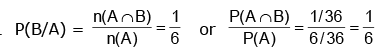Ex.14 A bag contains 3 red, 6 white and 7 blue balls. Two balls are drawn one by one. What is the probability that first ball is white and second ball is blue when first drawn ball is not replaced in the bag ?
Sol.
Let A be the event of drawing first ball white and B be the event of drawing second ball blue.
Here A and B are dependent events.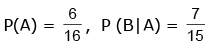⇒ P(AB) = P(A). P(B/A) =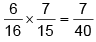Ex.15 A bag contains 4 red and 4 blue balls. Four balls are drawn one by one from the bag, then find the probability that the drawn balls are in alternate colour.
Sol.
E1 : Event that first drawn ball is red, second is blue and so on.
E2 : Event that first drawn ball is blue, second is red and so on.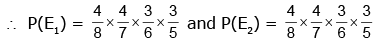⇒  P(E) = P(E1) + P(E2) =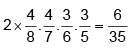J. BINOMIAL PROBABILITY DISTRIBUTION

Suppose that we have an experiment such as tossing a coin or die repeatedly or choosing a marble from an urn repeatedly. Each toss or selection is called a trial. In any single trial there will be a probability associated with a particular event such as head on the coin, 4 on the die, or selection of a red marble. In some cases this probability will not change from one trial to the next (as in tossing a coin or die.) Such trials are then said to be independent and are often called Bernoulli trials after James Bernoulli who investigated them at the end of the seventeenth century.

Let p be the probability that an event will happen in any single Bernoulli trial (called the probability of success). Then q = 1 – p is the probability that the event will fail to happen in any single trial (called the probability of failure). The probability that the event will happen exactly x times in n trials (i.e., n successes and n – x failures will occur) is given by the probability function.
where the random variable X denotes the number of successes in n trials and x = 0, 1, ........n.

Example : The probability of getting exactly 2 heads in 6 tosses of a fair coin is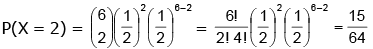The discrete probability function (i) is often called the binomial distribution since for x = 0, 1, 2, .........n, it corresponds to successive terms in the binomial expansion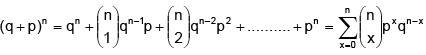The special case of a binomial distribution with n = 1 is also called the Bernoulli distribution.

Ex.18 If a fair coin is tossed 10 times, find the probability of

Sol.    The repeated tosses of a coin are Bernoulli trials. Let X denotes the number of heads in an experiment of 10 trials. Clearly, X has the binomial distribution with n = 10 and p =
Therefore     P(X = x) = nCxqn–x px, x = 0, 1, 2, ........n
Here n = 10, p = $$1 \over 2$$ , q = 1 – p = $$1 \over 2$$.

Therefore   P(X = x) =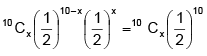Now (i)   P(X = 6) =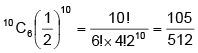(ii)    P (atleast six heads) = P (X ≥ 6) = P (X = 6) + P (X = 7) + P(X = 8) + P (X = 9) + P(X = 10)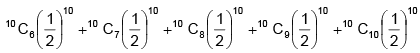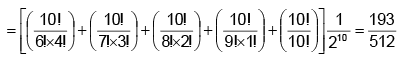(iii)    P (at most six heads) = P (X ≤ 6)
= P(X = 0) + P(x = 1) + P(X = 2) + P(X = 3) + P(X = 4) + P(X = 5) + P(X = 6)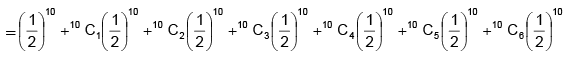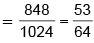Ex.19    A coin is tossed 7 times. Each time a man calls head. The probability that he wins the toss on more than three occasions is
Sol.
The man has to win at least 4 times then required probability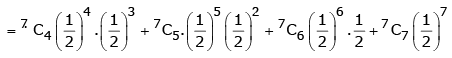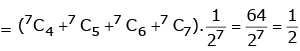Ex.20    A man takes a step forward with probability 0.4 and backward with probability 0.6. Find the probability that at the end of eleven steps he is one step away from the starting point.
Sol.
Since the man is one step away from starting point mean that either
(i) man has taken 6 steps forward and 5 steps backward.
(ii) man has taken 5 steps forward and 6 steps backward.
Taking, movement 1 step forward as success and 1 step backward as failure.
∴      p = Probability of success = 0.4 and q = Probability of failure = 0.6
∴  Required Probability = P [X = 6 or X = 5] = P [X = 6] + P(X = 5) =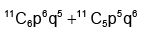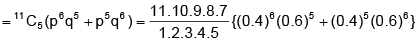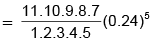Hence the required probability = 0.37

K. TOTAL PROBABILITY THEOREM Let an event A occurs with one of the n mutually exclusive & exhaustive events B1, B2, B3, ...... Bn
then A = AB+ AB2 + AB3 + ................+ABn

P(A) = P(AB1) + (PAB2 )+ (PAB3 )+ ................+P(ABn) =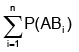P(A) = P(B1) P(A | B1) + P(B2) P(A | B2) + ......................+ P(Bn) P (A | Bn) =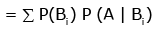Ex.21     A purse contains 4 copper and 3 silver coins and another purse contains 6 copper and 2 silver coins. One coin is drawn from any one of these two purses. The probability that it is a copper coin is
Sol.
Let  A ≡  event of selecting first purse
B ≡ event of selecting second purse
C ≡ event of drawing a copper coin
Then given event has two disjoint cases : AC and BC
∴     P(C) = P (AC + BC)  = P (AC) + P (BC) = P(A) P (C) + P(B) P(C) =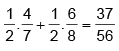Ex.22    Three groups A, B, C are contesting for positions on the Board of Directors of a Company. The probabilities of their winning are 0.5, 0.3, 0.2 respectively. If the group A wins, the probability of introducing a new product is 0.7 and the corresponding probabilities for group B and C are 0.6 and 0.5 respectively. Find the probability that the new product will be introduced.
Sol.
Given P(A) = 0.5, P(B) = 0.3 and P(C) = 0.2
∴  P(A) + P(B) + P(C) = 1 then events A, B, C are exhaustive.
If P(E) = Probability of introducing a new product, then as given
P(E | A) = 0.7, P(E | B) = 0.6 and P(E | C) = 0.5.
∴  P(E) = P(A) . P(E | A) + P(B). P(E | B) + P(C). P(E|C)
= 0.5 × 0.7 + 0.3 × 0.6 + 0.2 × 0.5 = 0.35 + 0.18 + 0.10 = 0.63

L. BAYE'S THEOREM

If an event A can occur only with one of the n mutually exclusive and exhaustive events B1. B2,......Bn & the probabilities P(A|B1), P(A|B2) ............... P(A|Bn) are known then  P(B1 | A) =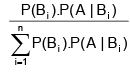Explanation :
A  ≡ event what we have ; B1 ≡ event what we want ;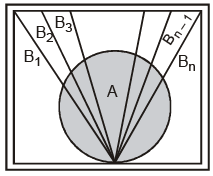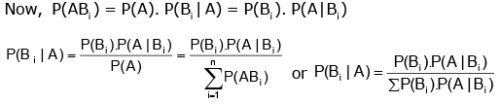Ex.23    Given three identical boxes I, II and III, each containing two coins, In box I,  both coins are gold coins, in box II, both are silver coins and in the box III, there is one gold and one silver coin. A person chooses a box at random and takes out a coin. If the coin is of gold, what is the probability that the other coin in the box is also of gold ?
Sol.
Let E1, E2 and E3 be the events that boxes I, II and III are chosen, respectively.
Then P(E1) = P(E2) = P(E3) = 1/3. Also, let A be the event that 'the coin drawn is of gold'
Then P(A | E1) = P (a gold coin from box I) =2/2) = 1
P(A | E2) = P (a gold coin from box II) = 1/2
Now, the probability that the other coin in the box is of gold
= the probability that gold coin is drawn from the box I = P (E1 | A)
By Baye's theorem,

P (E1| A) =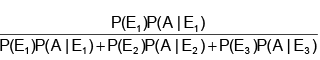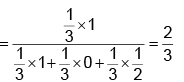Ex.24  In a factory which manufactures bolts, machines A, B and C manufacture respectively 25%, 35% and 40% of the bolts. Of their outputs, 5, 4 and 2 percent are respectively defective bolts. A bolt is drawn at random from the product and is found to be defective. What is the probability that it is
manufactured by the machine B ?
Sol.
Let events B1, B2, B3 be the following :

B1 : the bolt is manufactured by machine A
B: the bolt is manufactured by machine B
B3 : the bolt is manufactured by machine C
Clearly, B1, B2, B3 are mutually exclusive and exhaustive events and hence, they represents a partition of the sample space.
Let the event E be ' the bolt is defective. The event E occurs with B1 or with B2 or with B3.
Given that P(B1) = 25% = 0.25, P(B2) = 0.35 and P(B3) = 0.40
Again P(E | B1) = Probability that the bolt drawn is defective given that it is manufactured by machine A = 5% = 0.05. Similarly, P(E | B1) = 0.04, P (E | B3) = 0.02
Hence, by Bayes' Theorem, we have P(B2 | E) =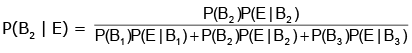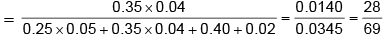M. PROBABILITY THROUGH STATISTICAL (STOCHATIC) TREE DIAGRAM

These tree diagrams are generally drawn by economist and give a simple approach to solve a problem.

Ex.25    A bag initially contains 1 red ball and 2 blue balls. A trial consists of selecting a ball at random noting its colour and replacing it together with an additional ball of the same colour. Given that three trials are made, draw a tree diagram illustrating the various probabilities. Hence or otherwise, find the probability that
(a) atleast one blue ball is drawn
(b) exactly one blue ball is drawn
(c) Given that all three balls drawn are of the same colour find the probability that they are all red.

Sol.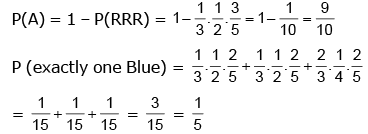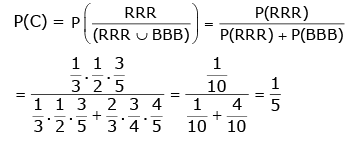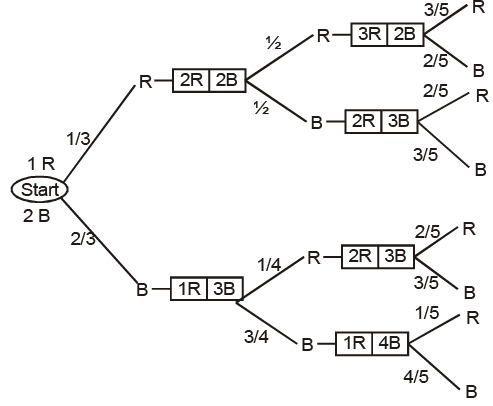N. COINCIDENCE TESTIMONY
If p1 and p2 are the probabilities of speaking the truth of two independent witnesses A and B then
P (their combined statement is true) =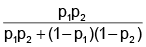In this case it has been assumed that we have no knowledge of the event except the statement made by A and B. However if P is the probability of the happening of the event before their statement then P (their combined statement is true) =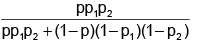Here it has been assumed that the statement given by all the independent witnesses can be given in two ways only, so that if all the witnesses tell falsehoods they agree in telling the same falsehood. If this is not the case and c is the change of their coincidence testimony then the  probability that the statement is true = p p1 p2
probability that the statement is false = (1 – p).c (1 – p1) (1 – p2)
However chance of coincidence testimony is taken only if the joint statement is not contradicted by any witness.

Ex.26    A speaks truth in 75% case and B in 80% cases. What is the probability that they contradict each other in stating the same fact ?
Sol.
There are two mutually exclusive cases in which they contradict each other. i.e.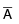B and A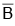Hence required probability =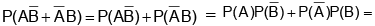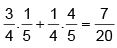O.  PROBABILITY DISTRIBUTION

(a)    A Probability Distribution spells out how a total probability of 1 is distributed over several values of a random variable.
(b)    Mean of any probability distribution of a random variable is given by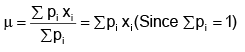(c)    Variance of a random variable is given by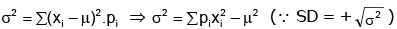(d)  The probability distribution for a binomial variate 'X' is given by ; P (X = r) = nCr pqn–r. The recurrence formula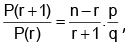, is very helpful for quickly computing P(1), P(2), P(3) etc. if P(0) is known.
(e)  Mean of BPD = np ; variance of BPD = npq.
(f) If p represents a persons chance of success in any venture and 'M' the sum of money which he will receive in case of success, then his expectations or probable value = pM expectations = pM

P. GEOMETRICAL PROBABILITY
The following statements are axiomatic :

(a)  If a point is taken at random on a given straight line AB, the chance that it falls on a particular segment PQ of the line is PQ/AB
(b)  If a point is taken at random on the area S which includes an area s, the chance that the points falls on s is s/S.

Offer running on EduRev: Apply code STAYHOME200 to get INR 200 off on our premium plan EduRev Infinity!

## Mathematics (Maths) Class 11

158 videos|187 docs|157 tests

,

,

,

,

,

,

,

,

,

,

,

,

,

,

,

,

,

,

,

,

,

;